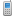MV Software Knowledge Bas… Modules»Inventory Control»Average Cost Calculation

Tags:

# Average Cost Calculation

To determine how the weighted average cost on an item is calculated, see the formula below.  When inventory items are vouchered through Vendor Invoice Entry & Editing (Task 3 in the Purchase Order module) the latest cost can be updated on the Inventory Master and Supplier Master records.  A parameter determines if the cost should be updated or not.  The parameter, found in 7/32/22/3, is: DFLT'CHG'FLG

Formula:   [(previous quantity times average cost) plus (purchased quantity times unit cost)] divided by the total quantity (previous quantity plus purchased quantity).

NOTE:   IF the quantity on an item goes to less than zero (a negative quantity), then the new Last Cost  becomes the new Average Cost.  The ‘formula’ at that point is not used at all.

i.e.        previous quantity =       12

average cost =              \$1.00

previous qty x average cost =                12.00

purchased quantity =     20

purchased unit cost =    \$1.20

purchqty x purch.unit cost =                \$24.00

\$12.00 + \$24.00 =                               \$36.00

previous quantity =       12

purchased quantity =     20

prev. qty + purch. qty =                        32\$36.00 divided by 32 =                        \$1.125 or \$1.13  new average cost

[(prev qty * avg)      + (purch qty * unit cost)] divided by (prev qty + purch qty).

12     *   \$1.00    +       20      *   \$1.20           /            12        +       20

\$12.00  +  \$24.00 = \$36.00 divided by 32 =  \$1.125 or \$1.13

12 x  \$1.00      =          \$12.00

20 x  \$1.20      =          \$24.00

\$36.00   / (12 + 20)  32   =    \$1.125 or \$1.13